#### 期刊菜单

230 kV亚马逊河大跨越工程跨越段导线防振创新要点
Innovation of Anti-Vibration Scheme for Overhead Conductor of the Crossing Section in 230 kV Amazon River Crossing Project
DOI: 10.12677/SG.2022.123009, PDF, HTML, XML, 下载: 157  浏览: 190

Abstract: In order to solve the difficult problem of conductor anti-vibration caused by large tension and single conductor in Amazon River crossing project. The method of dividing the span into two sections and design anti-vibration scheme separately is proposed. The energy balance method is used to process the test data, which proves the advantages of the method proposed in this paper over the traditional method. The new method is of great significance for the anti-vibration of conductors with large ratio of steel area to aluminum-alloy area.

1. 引言

2. 工程信息和试验

2.1. 工程信息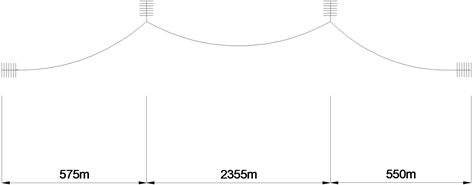Figure 1. Crossing span s of the project

Table1. Conductor parameters

2.2. 试验系统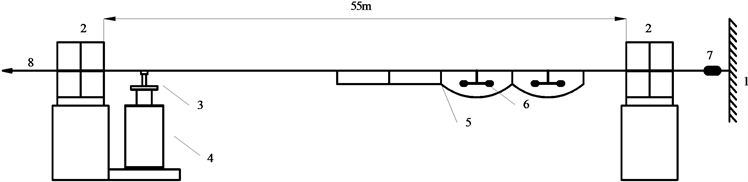1 固定端2 重型线夹3 连接金具4 振动台5 Bate阻尼线6 防振锤 7 力传感器8 张拉端

Figure 2. Schematic diagram of test device

2.3. 传感器布置

2.4. 试验操作

${P}_{t}=\frac{1}{2}\cdot F\cdot V\cdot \mathrm{cos}\phi$ (1)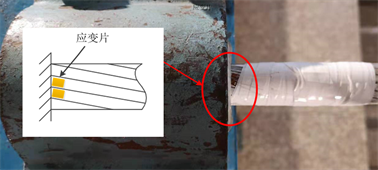Figure 3. Strain sensors on the conductor at the end

1) 以15 Hz的振动频率激振架设好的导线，利用振动台向导线输入五个不同的能量(按从小到大的顺序，如2 W、4 W、8 W、16 W和32 W等)，使导线产生不同强度的振动；

2) 记录不同振动强度下导线的振幅和各个线夹夹固点的应变；

3) 改变导线振动频率并重复(1)~(2)操作步骤。频率变化步长约为5 Hz，直至频率为65 Hz左右；

4) 整理功率、振幅和应变数据，待下一步使用。

3. 试验分析方法和试验结果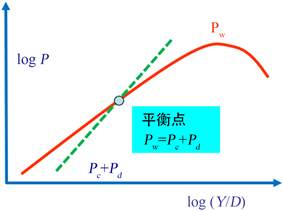Figure 4. The sketch of energy balance method

3.1. 导线自阻尼特性

${P}_{c}=\Phi \left(f,Y\right)={10}^{\beta }{\left(Y/D\right)}^{\alpha }$ (2)

$\mathrm{log}{P}_{c}=\alpha \mathrm{log}\left(Y/D\right)+\beta$ (3)

$\alpha =2.151141+0.010837f$ (4)

$\beta =1.508353+0.059485f$ (5)

$f=K\frac{v}{d}$ (6)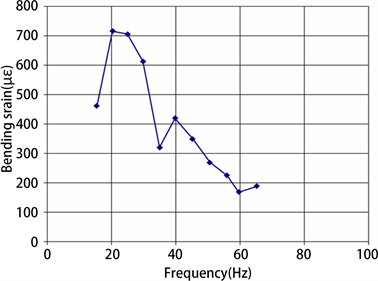Figure 5. Self-damping characteristics of conductor AACSR/UGS 540

3.2. 导线系统特性

${P}_{t}={P}_{c}=\Phi \left(f,Y\right)={10}^{\beta }{\left(Y/D\right)}^{\alpha }$ (7)

${P}_{t}={P}_{c}+{P}_{d}=\Phi \left(f,Y\right)={10}^{\beta }{\left(Y/D\right)}^{\alpha }$ (8)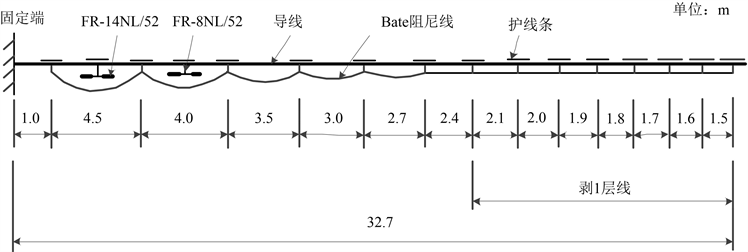Figure 6. Initial combined anti-vibration device diagram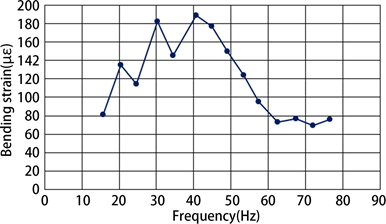Figure 7. Frequency response of the conductor with initial combined damping device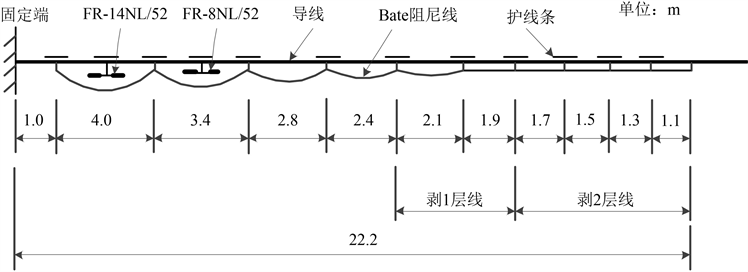Figure 8. Diagram of combined anti-vibration device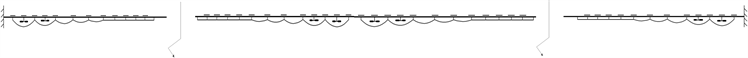Figure 9. The final anti-vibration scheme of crossing section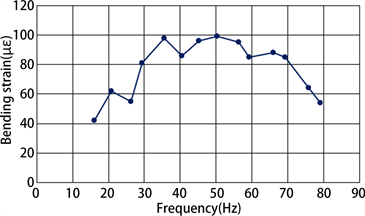Figure 10. The frequency response of conductor with final anti-vibration scheme

4. 结论

  张昭, 周立宪, 齐翼, 等. 大跨越导线微风振动强度影响因素[J]. 振动、测试与诊断, 2021, 41(4): 710-714.  郑玉琪. 架空输电线微风振动[M]. 北京: 水利电力出版社, 1987.  万建成. 架空导线应用技术[M]. 北京: 中国电力出版社, 2015: 62-65.  (2013) IEC TC 7. IEC 62567-2013 Methods for Testing Self-Damping Characteristics of Conductors. IEC, Geneva.  de Miranda, T.B., Kalombo, R.B. and Araújo, J.A. (2019) Self-Damping of AAC, AAAC and ACSR Conductors with Respect of H/W Parameter. 25th ABCM International Congress of Mechanical Engineering, Uberlândia, 20-25 October 2019, 2-9. https://doi.org/10.26678/ABCM.COBEM2019.COB2019-1666  张昭, 齐翼, 张暕, 等. 特高压交流工程用套环对导线微风振动的影响[J]. 噪声与振动控制, 2020, 40(6): 245-249.  刘胜春, 王景朝, 吴建生, 等. 500 kV大跨越四分裂导线及地线防振试验研究[J]. 振动、测试与诊断, 2007, 27(4): 332-336.  齐翼, 芮小明, 尹泉, 等. 特高压直流工程用大截面导线的阻尼特性[J]. 噪声与振动控制, 2019, 39(1): 201-204.  张殿生. 电力工程高压送电线路设计手册[M]. 北京: 中国电力出版社, 2003: 219.  中国电力企业联合会. GB 50545-2010 110 kV~750 kV架空输电线路设计规范[S]. 北京: 中国计划出版社, 2010.